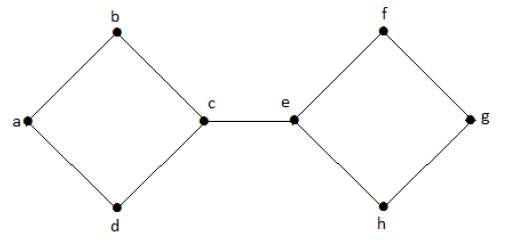# Graph Theory Problem-Test Series

1.1k views
A Connected Graph has Cut edge, Then Graph has Cut vertex also.

1. True

2. False

Choose Correct One.

True.

If a graph has a cut edge then there is definitely a cut vertex but vice versa is not true.selected
0
can u give example of viceversa ?
0

## Cut Vertex

Let ‘G’ be a connected graph. A vertex V ∈ G is called a cut vertex of ‘G’, if ‘G-V’ (Delete ‘V’ from ‘G’) results in a disconnected graph. Removing a cut vertex from a graph breaks it in to two or more graphs.

Note − Removing a cut vertex may render a graph disconnected.

A connected graph ‘G’ may have at most (n–2) cut vertices.

### Example

In the following graph, vertices ‘e’ and ‘c’ are the cut vertices.By removing ‘e’ or ‘c’, the graph will become a disconnected graph.

## Cut Edge (Bridge)

Let ‘G’ be a connected graph. An edge ‘e’ ∈ G is called a cut edge if ‘G-e’ results in a disconnected graph.

If removing an edge in a graph results in to two or more graphs, then that edge is called a Cut Edge.

### Example

In the following graph, the cut edge is [(c, e)]By removing the edge (c, e) from the graph, it becomes a disconnected graph.

0
I don't think it is true to consider that a graph having a cut edge will definitely have cut vertex.

Consider a graph with a single edge A------B, if we remove this edge graph will get disconnected but if we remove vertex A, a graph will be connected as a graph with the single vertex is still consider connected.

## Related questions

1
718 views
The Chromatic Number of Cycle Graph with 7 vertices _____
How to PROVE S2 is correct?? Consider the statements $S_1$ ) In any simple graph with more than one vertex, there must exist at-least $2$ vetices of the same degree $S_2$ ) A graph with $13$ vertices, $31$ edges, $3$ vertices of degree $5$ and $7$ vertices of degree $4$ does not ... true B). $S_1$ is true and $S_2$ is false C). $S_1$ is false and $S_2$ is true D). Both $S_1$ and $S_2$ are true